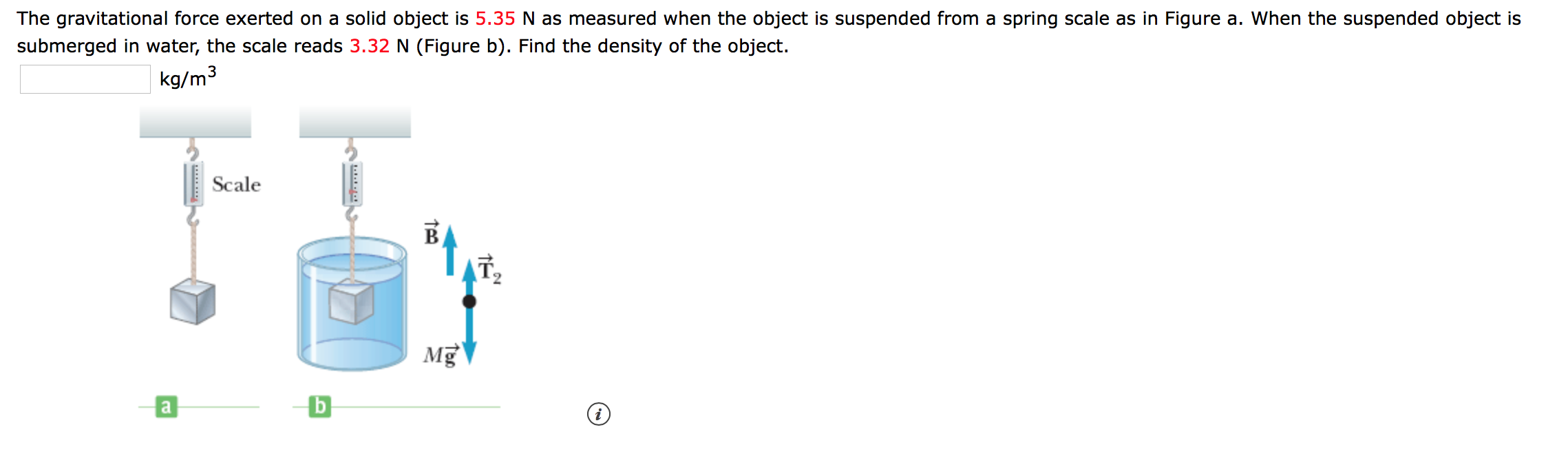# The gravitational force exerted on a solid object is 5.35 N as measured when the object is suspended from a spring scale as in Figure a. When the suspended object is submerged in water, the scale reads 3.32 N (Figure b). Find the density of the object. kg/m3 Scale b a Tao

Questionhelp_outlineImage TranscriptioncloseThe gravitational force exerted on a solid object is 5.35 N as measured when the object is suspended from a spring scale as in Figure a. When the suspended object is submerged in water, the scale reads 3.32 N (Figure b). Find the density of the object. kg/m3 Scale b a Tao fullscreen